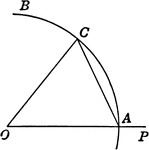### Construction of Angle

Illustration of construction to lay off an angle.### Circle with Arc, Chord, Inscribed Angle, Circumscribed Polygon

Illustrations of a circle with arc, chord, inscribed angle, and circumscribed about a polygon.### Arc and Chord in Circle

Illustration of circle with arc and chord.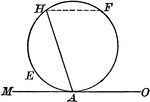### Circle With Chord and Tangent

Illustration of a circle showing an angle included by a tangent and a chord drawn from the point of…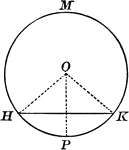### Circle with Chord and Triangle

Illustrations of a circle with a chord and triangle inside.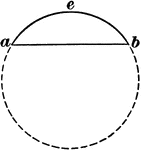### Chord of Circle

Illustration of a circle with the chord eb drawn and labeled.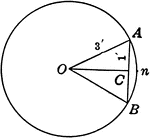### Circle With a Chord of 2 ft. and a Radius of 3 ft.

Circle with chord AB=2 ft. and radius OA = 3 ft.. Triangle AOC is a right triangle. Angle AOC=half angle…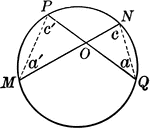### Circle With Intersecting Chords

Circle with intersecting chords.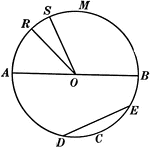### Chords, Diameters, and Radii of a Circle

Illustration of a circle with diameter AB. Radii are RO, SO, AO, and BO. ED is a chord.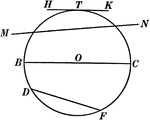### Chords, Secants, Diameters, and Tangents of a Circle

Illustration of a circle with diameter BC, chord DF, secant MN, and tangent HK.### Circle With Diameter and Chord

Illustration of a circle with diameter and chord drawn. A diameter perpendicular to a chord bisects…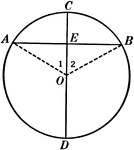### Diameter Perpendicular to a Chord in a Circle

Illustration used to show that "The diameter perpendicular to a chord bisects the chord and also its…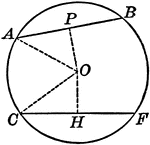### Circle With Equal Chords

Illustration of a circle with equal chords, which are equally distant from the center.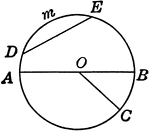### Circle With Parts

Circle with diameter, radius, arc, chord, and arc.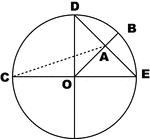### Parts of Circle

Illustration of circle with arc, chord, diameter and radius.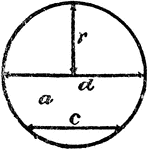### Radius, Diameter, and Chord In A Circle

An illustration showing a circle with radius r, diameter d, and chord c.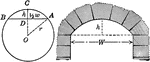### Segments of Circle and Arch

Illustration of circle with segments labeled and arch.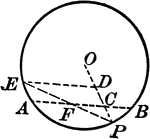### Circle and Triangle

Circle with triangle and chords.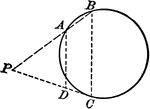### Circle and Triangle

Circle with triangle and chords.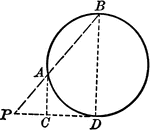### Circle and Triangle

Circle with triangle and chords.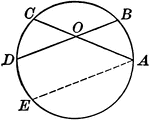### Circle With Two Intersecting Chords

Illustration of a circle with two intersecting chords within the circumference. The angle formed is…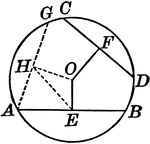### Circle With Unequal Chords

Illustration of a circle with unequal chords, which are unequally distant from the center.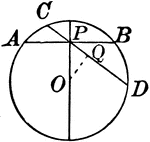### Circle With Various Chords

Illustration showing a circle with various chords drawn.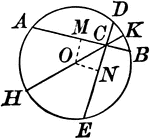### Circle With Various Chords

Illustration showing a circle with various chords drawn.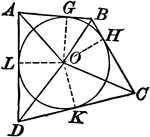### Circle With Various Chords And Tangents

Illustration showing a circle with various chords and tangents drawn.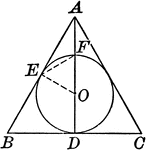### Circle With Various Chords And Tangents

Illustration showing a circle with various chords and tangents drawn.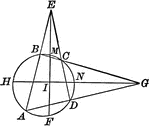### Circle With Inscribed Quadrilateral and Triangles Formed

Illustration showing a circle with an inscribed quadrilateral and triangles formed by extended chords.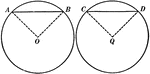### Equal Chords in Equal Circles Theorem

Illustration used to show that "In equal circles, or in the same circle, if two chords are equal, they…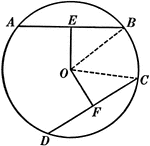### Equal Chords in Equal Circles Theorem

Illustration used to show that "In equal circles, or in the same circle, if two chords are equal, they…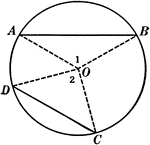### Unequal Chords in Circles Theorem

Illustration used to show that "In equal circles, or in the same circle, if two chords are unequal,…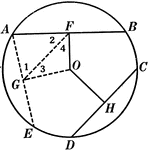### Unequal Chords in Equal Circles Theorem

Illustration used to show that "In equal circles, or in the same circle, if two chords are unequal,…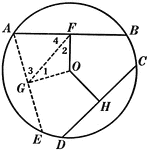### Unequal Chords in Equal Circles Theorem

Illustration used to show that "In equal circles, or in the same circle, if two chords are unequal,…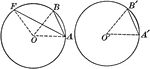### Equal Circles With Radii and Chords

Illustrations of 2 equal circles with radii drawn.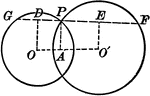### Intersecting Circles

Intersecting circles with chords and radii.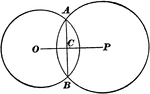### Intersecting Circles

Illustration of intersecting circles. "If two circles intersect each other, the line joining their centers…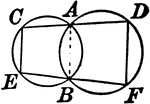### Two Intersecting Circles With Lines

Illustration showing 2 intersecting circles with a line drawn through each point of intersection terminated…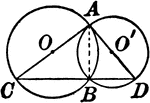### Two Intersecting Circles With Lines

Illustration showing 2 intersecting circles with a lines drawn that form a triangle.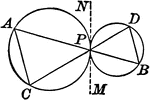### Tangent Circles With Chords

Illustration showing 2 circles with that touch each other and two lines drawn through the point of contact…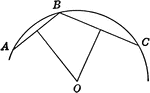### Construction of Arc When Given the Chord and Height of the Segment

Illustration of of construction of an arc when the chord and height of the segment are given.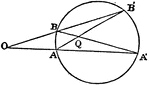### Radical Center of 3 Circles

Given any three circles, the common chords meet at one point.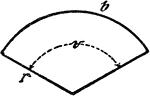### Circle Sector

An illustration showing a circle sector with radius r, center/central angle v, and length of circle…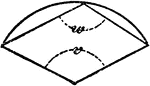### Circle Sector

An illustration showing a circle sector with center/central angle v and polygon angle w.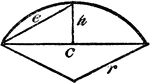### Circle Sector

An illustration showing a circle sector with height of segment h and radius r.### Semicircle With Chords and Radii

Illustration of a semicircle with chords and radii.